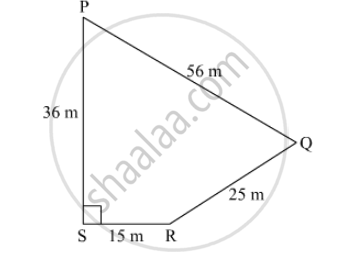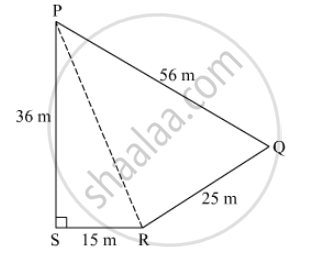Advertisement Remove all ads

# Look at the Measures Shown in the Adjacent Figure and Find the Area of ☐ Pqrs. - Mathematics

Sum

Look at the measures shown in the adjacent figure and find the area of ☐ PQRS.Advertisement Remove all ads

#### SolutionJoin PR.
In triangle PSR,
Applying Pthygoras theorem,

PS²  + SR²  = PR²

⇒ 36²  + 15²  = PR²

⇒ PR²  = 1296 + 225 = 1521

⇒ PR = sqrt = 39 m

In triangle PQR,

s = (56 + 25 + 39)/2 = 60

Area =sqrt[60 ( 60 - 56) (60 -25) (60 -39)]

= sqrt[60 xx 4 xx 35 xx 21]

= sqrt

= 420 m²

Area of triangle PSR = 1/2 xx 15 xx 36 = 270 m²
Area of PQRS = Area of triangle PSR + Area of triangle PQR
= 270 + 420
= 690 m2

Is there an error in this question or solution?
Advertisement Remove all ads

#### APPEARS IN

Balbharati Mathematics 8th Standard Maharashtra State Board
Chapter 15 Area
Practice Set 15.4 | Q 2 | Page 101
Advertisement Remove all ads
Advertisement Remove all ads
Share
Notifications

View all notifications

Forgot password?